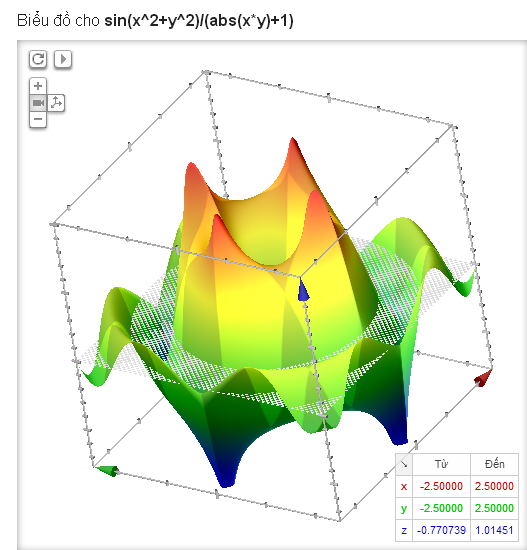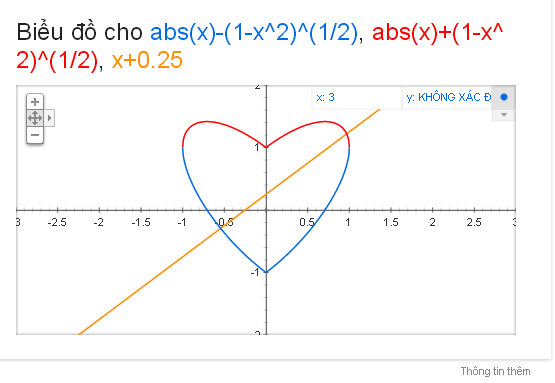## Jan 13, 2014

Như ta đã biết, công nghệ WebGL đã được Google bổ sung vào trình duyệt Chrome cách đây khá lâu cho phép bạn có thể trải nghiệm các nội dung giàu hình ảnh 3D phức tạp trong Google Chrome mà không cần cài đặt phần mềm bổ sung bên ngoài. Kết hợp với WebGL, Google hiển thị đồ thị toán học dưới dạng 3D vô cùng trực quan khi bạn tìm kiếm 1 hàm nào đó. Với óc sáng tạo độc đáo của người dùng, họ đã sử dụng Google Search tạo ra nhiều đồ thị đẹp mắt.Sau đây mình xin giới thiệu một số hình ảnh tiêu biểu.Xem linkXem linkXem link

Sau đây là một số đồ thị khác mà mình sưu tập được:

sin(x^2+y^2)/(abs(x*y)+1) from -2.5 to 2.5 [link]
sin(5.5x)*cos(5*y)+x*x+1 x is from -1 to 1, y is from -1 to 1, z is from 0.1 to 2.8 [link]
100-3/(sqrt(x^2+y^2))+sin(sqrt(x^2+y^2)), x is from -10 to 10, y is from -10 to 10, z is from 85 to 101 [link]
5 + (-sqrt(1-x^2-(y-abs(x))^2))*cos(30*((1-x^2-(y-abs(x))^2))), x is from -1 to 1, y is from -1 to 1.5, z is from 1 to 6 [link]
100-3/(sqrt(x^2+y^2))+sin(sqrt(x^2+y^2))+sqrt(200-(x^2+y^2)+10*sin(x)+10sin(y))/1000, x is from -15 to 15, y is from -15 to 15, z is from 90 to 101 [link]
exp(-((x-4)^2+(y-4)^2)^2/1000) + exp(-((x+4)^2+(y+4)^2)^2/1000) + 0.1exp(-((x+4)^2+(y+4)^2)^2)+0.1exp(-((x-4)^2+(y-4)^2)^2) [link]
sqrt(cos(3*x))*cos(100*y)+1.5*sqrt(abs(x)) + 0.8 x is from -1 to 1, y is from -1 to 1, z is from 0.01 to 2.5 [link]
sqrt(x*y+y*y)+3*sin(sqrt(x*x+y*y))+5 from -20 to 20 [link]
x^2+y^2+x*y*sin(x+y) from -20 to 20 [link]

WebGL.com

1.2.